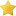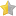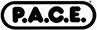# Linear Regression Analysis (Online CE Course)(based on 187 customer ratings)

How to Subscribe
 MLS & MLT Comprehensive CE PackageIncludes 173 CE courses, most popular \$95 Pick Your CoursesUp to 8 CE hours \$50 Individual course \$20
Need multiple seats for your university or lab? Get a quote

The purpose of this course is to demonstrate how to use linear regression to predict the value of one variable, given the value of the other variable and the experimental data concerning the relationship between the variables.

See all available courses

### Continuing Education CreditsP.A.C.E.® Contact Hours (acceptable for AMT, ASCP, and state recertification): 2.5 hour(s)Approved through 5/31/2024Florida Board of Clinical Laboratory Personnel Credit Hours - Supervision/Administration, Quality Control/Quality Assurance, and Safety: 2.5 hour(s)Approved through 5/31/2024

### Objectives

• Define linear regression and explain how it is used.
• Given data points that fall on a straight line, find the equation for the line.
• Use the regression equation to predict the value of a dependent variable give the value of the independent variable.
• Explain what is meant by the phrase "line of best fit."
• Given a set of data, determine the best fit using the least squares method.
• Define and calculate standard error of estimate.
• Explain the difference between a, alpha, b, and beta, as applied to regression analysis, and describe why confidence intervals are calculated for the slope and y-intercept.

### Customer Ratings(based on 187 customer ratings)

### Course Outline

Click on the links below to preview selected pages from this course.
• Introduction to Regression Analysis
• Predicting a Value
• A Regression Analysis Example
• A Regression Analysis Example, continued
• Calculating the Y-Intercept
• Prediction Using the Resulting Equation
• Given the following creatinine standards:mg/dLAbsorbance30.1460.2690.38What is the correct form of the regression line?
• Given the data and linear regression line you calculated on the previous question, what is the expected absorbance of a 10 mg/dL sample?
• True or false: you should make a scatterplot of your data before you calculate the regression line.
• A scatterplot of the data below is shown on the right, confirming a linear relationship. Given the following data, calculate the regression line.xy2 9...
• Introduction to Least Squares Method of Best Fit
• Introduction to Least Squares Method
• The Least Squares Line
• Standard Error of Estimate
• Calculate the sum of squares for line B. To do this, you must calculatethe difference y- , and the squared difference (y-)2 for each point, and then s...
• Using the sum of squares from the previous question (440.25), calculate the Standard Error of Estimate for line B to the nearest thousandth using the ...
• Least Squares Calculation
• Determining the Least Squares Line
• Formulae for Determining the Slope and Intercept
• Calculating the Standard Error of Estimate
• Correlation Coefficient
• Example Regression Line Calculation
• Using the Least Squares Formulae
• Determining Se and r2
• Data for Questions
• Using the data, calculate the total of the (x-)(y-) values. What is the total (rounded to the nearest whole number)?PointRef. Method (x)Test Method (y...
• Using the same data, calculate the total of the (x-)2 values. What is the total?The average of x is 20 and the average of y is 23.8.PointRef. Method (...
• Using the formulas below and the information from the previous questions (shown again in the table below), what are the slope and y-intercept of the l...
• What is the Standard Error of Estimate for this regression line, using the shortcut form of the equation shown below:a = 2.4b = 1.070= 23.8PointRef. M...
• Calculation of Confidence Intervals for Least Squares
• References
• References

Level of instruction: Intermediate
Intended audience: This course is appropriate for laboratory professionals, and for students in clinical laboratory science programs who want a review of the statistics that are analyzed for assessment of quality control.
Author Information:  Mary Ann Fiene, MT(ASCP), has authored several articles on the subjects of curriculum development, competency evaluation, and job restructuring. Her articles have appeared in the Journal of Allied Health, American Journal of Medical Technology (now published as Clinical Laboratory Science), and Medical Laboratory Observer. Ms. Fiene was affiliated as an educator with the Kettering Medical Center School of Medical Technology.
Alan Reichert, PhD, is a professor of finance at Cleveland State University in Ohio.
Reviewer Information: Alexandru Casapu, MBA, MLS(ASCP)CM, PBTCM has over 20 years of experience as a medical laboratory scientist, section supervisor, and laboratory manager. He is the former Director of Clinical Laboratory Technology Program at Georgia Piedmont Technical College. He is currently a Program Director at MediaLab, Inc. Alexandru holds BS degrees in Biology and Medical Technology from Clark Atlanta University and an MBA from the University of Georgia.
About the Course: This course is part of a series of courses adapted for the web by MediaLab Inc. under license from Educational Materials for Health Professionals Inc. Dayton OH, 45420. Copyright EMHP. The course was reviewed and revised in 2018.

### Keywords

These are the most common topics and keywords covered in Linear Regression Analysis:

How to Subscribe
 MLS & MLT Comprehensive CE PackageIncludes 173 CE courses, most popular \$95 Pick Your CoursesUp to 8 CE hours \$50 Individual course \$20
Need multiple seats for your university or lab? Get a quote